# Problem 57949. Easy Sequences 106: Counting Pythagorean Triangles inside a Circle

Pythagorean Triangle is a right triangle all sides of which are represented by integers. Examples are triangles with sides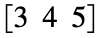and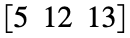.
Our goal in this exercise is to write a function that outputs the maximum number of unique pythagorean triangles that can fit inside a circle of radius R, with overlaps are allowed.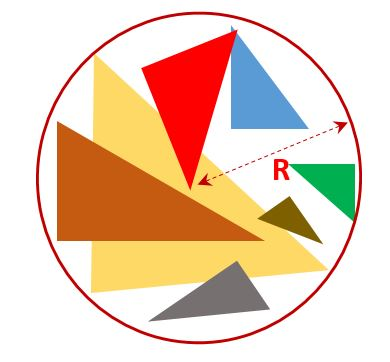For example if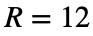, the following unique pythagorean triangles can fit inside the circle:,,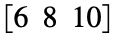,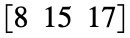,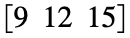, and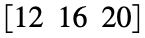. Hence, for, the function should return 6.
--------------------
NOTE: Unique means that congruent triangles, even if rotated or reflected, are counted only once.

### Solution Stats

50.0% Correct | 50.0% Incorrect
Last Solution submitted on Jun 12, 2023

### Community Treasure Hunt

Find the treasures in MATLAB Central and discover how the community can help you!

Start Hunting!